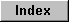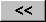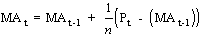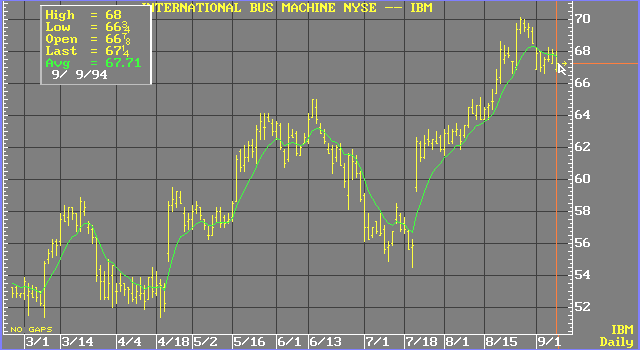# Modified Moving Averages

Modified moving averages are similar to simple moving averages. The first point of the modified moving average is calculated the same way the first point of the simple moving average is calculated. However, all subsequent points are calculated by first adding the new price and then subtracting the last average from the resulting sum. The difference is the new point, or modified moving average.

Formula:Where:

n = number of bars

MAt = current moving average value

MAt-1 = previous moving average value

Pt = current priceThis method is convenient because it is not necessary to keep track of all past components of the average. Only the last moving average value and the new price are necessary for the calculation (keep in mind that study calculations used to be done by hand). Because of the simplicity of this calculation, the modified moving average is used extensively for internal calculations in other analyses.

see, P. J. Kaufman, The New Commodity Trading Systems and Methods, New York: John Wiley & Sons, 1978, pp. 58-64.